## Monday, February 07, 2005

### Lagrange multipliers: equality constraints

Constrained optimization often comes up in machine learning.

1. Finding lengths of best instantaneous code when distribution of source is known
2. Finding maximum entropy distribution subject to given constraints
3. Minimizing distortion specified by the amount of information X provides about Y (Tishby's, Information Bottleneck method)
All of the examples above can be solved using the method of Lagrange multipliers. To understand motivation for Lagrange multipliers with equality constraints, you should check out Dan Klein's excellent tutorial.

However, you don't have to understand them to be able to go through the math. Here's how you can do it in 5 easy steps:

Write down the thing you are optimizing as J(x)
1. Write down your constraints as g_i(x)=0
2. Define L(x,l1,l2,...)=J(x) + l1 g1(x) + l2 g2(x) +...
3. Differentiate L with respect to each variable and set to 0
4. Solve this system of equations

Small Example

Suppose you want to maximize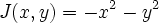subject to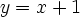Your problem looks like this: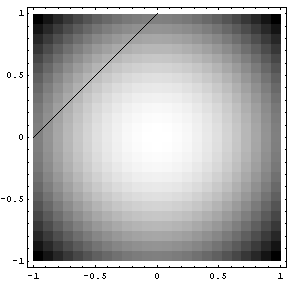(black line is where you solution must lie)

Then our only constraint function is g(x,y)=x-y+1=0. Our L (Langrangian) then becomes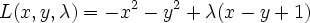differentiating and setting to 0 we get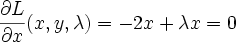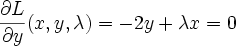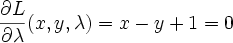Solve this system of 3 equations by substitution and you get x=-0.5, y=0.5, lambda=-1. The lagrange multiplier lambda_i represents ratio of the gradients of objective function J and i'th constraint function g_i at the solution point (that makes sense because they point in the same direction)

Bigger Example

For a more realistic example, consider maximizing entropy over a set of discrete distributions over positive integers where the first moment is fixed. Since we have an infinite number of possible X's, differentiating the Lagrangian gives us an countably infinite system of equations. But that's not actually a problem (unless you try to write them all down). Here's the detailed derivation

http://yaroslavvb.com/research/reports/fuzzy/fuzzy_lagrange.ps

So you can see that highest entropy discrete distribution with fixed mean is geometric distribution. We can solve the same problem for continuous X's in the positive domain, and get exponential distribution. If we allow X's to span whole reals, there will be no solution for the same reason as why "uniform distribution over all reals" doesn't exist.

Questions:

1. What to use for complicated 3d graphs? I tried Mathematica, but not sure how to combine two 3d graphs into one
2. Can anyone do Exercise 1. in Chapter 5 of Christianini's SVM book?
3. If we use Robinson's non-standard Analysis, could we sensibly define "uniform distribution over all integers"?

Resources: